#### Negative Feedback, Physics tutorial

Introduction:

Feedback is a procedure of transferring energy from the high-level point in the system to low-level point in the system. This signifies transferring energy from output of the amplifier back to its input. If output feedback signal opposes input signal, signal is degenerative or negative feedback.

If feedback signal is of opposite polarity to input signal, negative feedback results.

Amplifier gain with negative feedback is given by, A' = A/(1 + βA)

Obviously, A' < A because |1 + βA|>1

Suppose, A = 90 and β = 1/10 = 0.1

Then, gain without feedback is 90 and with negative feedback is

A' = A/(1 + βA) = 90/(1 + 0.1 x 90) = 9

Negative feedback decreases amplifier gain. That is why it is known as degenerative feedback. A lot of voltage gain is sacrificed because of negative feedback. When |βA|>>1, then

A' = A/βA ≈ 1/β

It signifies that A´ depends only on β. But it is very stable as it is not affected by changes in temperature, device parameters, and supply voltage and from aging of circuit components etc. As resistors can be selected accurately with almost zero temperature-coefficient of resistance, it is possible to attain highly precise and stable gain with negative feedback.

Benefits of Negative Feedback:

While negative feedback results in reduced overall voltage gain, various improvements are achieved, among them being:

• High input impedance
• Better stabilized voltage gain
• Improved frequency response
• Lower output impedance
• Reduced noise
• More linear operation
• Less harmonic distortion
• Less phase distortion
• Less amplitude distortion.

Gain Stability:

In addition to β factor setting a precise gain value, we are also interested in how stable feedback amplifier is compared to the amplifier is compared to amplifier without feedback.

Gain of amplifier with negative feedback is given by A' = A/(1 + βA)

If βA >> 1, then above expression is:

dA'/A' = (1/βA).(dA/A)

Decreased Distortion:

Let harmonic distortion voltage generated within amplifier change from D to D´ when negative feedback is applied to amplifier. Assume

D' = xD

Fraction of output distortion voltage that is feedback to input is

βxd

Therefore, new distortion voltage D´ that appears in output is

D' = D - βxDA

D' = D/(1 + βA)

It is obvious from above equation that D' < D. In fact, negative feedback decreases amplifier distortion by amount of loop gain i.e. by the factor of (1+βA).

Feedback over Several Stages:

Multistage amplifiers are utilized to attain greater voltage or current amplification or both. In this, we have choice of applying negative feedback to enhance amplifier performance. Either we apply some feedback across each stage or we can put it in one loop across whole amplifier.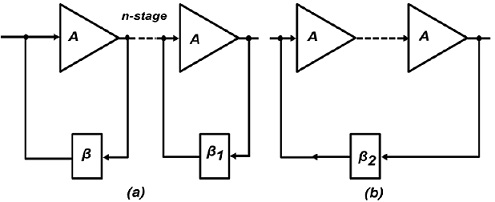In each stage of n-stage amplifier has feedback applied to it. Let A and β1 be open-loop gain and feedback ratio respectively of each stage and A1 overall gain of amplifier. The arrangement is where n amplifiers have been cascaded to get total gain of An. Let overall feedback factor be β2 and overall gain A2. Values of two gains are given as

A1 = (A/(1 + Aβ1)n and A1 = (An/(1 + Anβ2))

Differentiating above two expressions,

dA1/A1 = n(1 + Aβ1).(dA/A) and dA2/A2 = [n/(1 + Anβ2)].dA/A

For two circuits to have same overall gain, A1 = A2. Therefore, from equation

(dA2/A2)/(dA1/A1) = 1/((1 + aβ)n-1)

If n = 1, then denominator in above equation becomes unity so that fractional gain variations are same as expected. Though, for n > 1 and with (1+Aβ1) being normally large quantity, expression dA2/A2 will be less than dA1/A1. It signifies that overall feedback would appear to be advantageous as far as stabilizing of gain is concerned.

Forms of Negative Feedback:

The four basic arrangements for using negative feedback are explained below.

a) Voltage-series Feedback: it is also known as shunt-derived series-fed feedback. Amplifier and feedback circuit are joined series-parallel. Fraction of output voltage is applied in series with input voltage via feedback. The input to feedback network is in parallel with output of amplifier. Thus, so far as is concerned, output resistance of amplifier is decreased by shunting effect of input to feedback network. It can be proved that:

R'0 = R0/(1 + βA)

Series feedback always increases input impedance by the factor of (1 + βA).

b) Voltage-shunt Feedback: It is also called as shunt-derived shunt-fed feedback that is it is parallel-parallel (PP) prototype. Here, the small portion of output voltage is coupled back to input voltage parallel (shunt).

As feedback network shunts both output and input of amplifier, it decreases both its output and input impedances by the factor of 1/(1+ βA). A shunt feedback always decreases input impedance.

c) Current-series Feedback: It is also called as series-derived series-fed feedback. It is a series-series (SS) circuit. A part of output current is made to feedback a proportional voltage in series with input. As it is a series pick-up and series feedback, both input and output impedances of amplifier are increased because of feedback.

d) Current-shunt Feedback: It is also called as series-derived shunt-fed feedback. It is parallel-series (PS) prototype. Here, feedback network picks up part of output current and develops feedback voltage in parallel (shunt) with input voltage. As seen, feedback network shunts input but is in series with output. Therefore, output resistance of amplifier is increased whereas its input resistance is decreased by factor of loop gain.

Shunt-Derived Series-Fed Voltage Feedback:

The basic principle of such a voltage-controlled feedback is shown by block diagram. Feedback voltage is derived from voltage divider circuit formed of R1 and R2.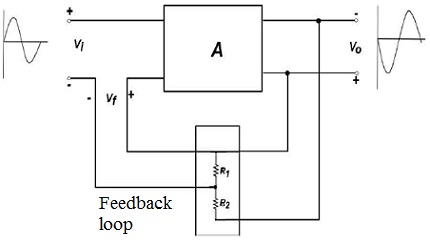As seen, voltage drop across R1 forms feedback voltage Vf.

Vf = V0(R1/(R1 + R2)) = βV0

Current-Series Feedback Amplifier:

As emitter resistor is un bypassed, it effectively gives current- series feedback. When IE passes through RE, feedback voltage drop Vf = IERE is developed that is applied in phase opposition to input voltage Vi.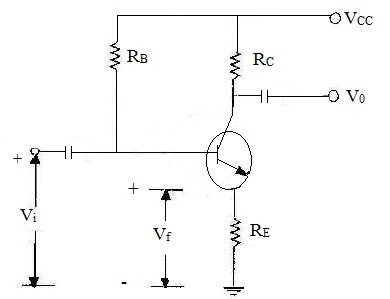This negative feedback decreases output voltage VC. This feedback can, though, be eliminated by either removing or bypassing emitter resistor. It can be proved that:

β = RE/RC; A' = RC/(re + RE); A = RC/re

Voltage-Shunt Negative Feedback Amplifier:

The portion of output voltage is coupled through Re in parallel with input signal at base. This feedback stabilizes overall gain while decreasing both input and output resistances. It can be that proved β = RC/RF.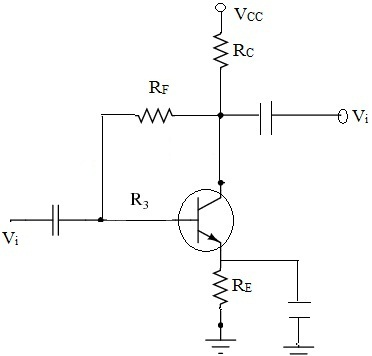Current-Shunt Negative Feedback Amplifier:

The feedback circuit (comprising of CF and RF) samples output current and develops the feedback voltage in parallel with input voltage. Unbypassed emitter resistor of Q2 gives current sensing. Polarity of feedback voltage is such that it gives the negative feedback.

Non-Inverting OP-AMP with Negative Feedback:

The input signal is applied to non-inverting input terminal. Output is applied back to input terminal through feedback network formed by Ri and Rf.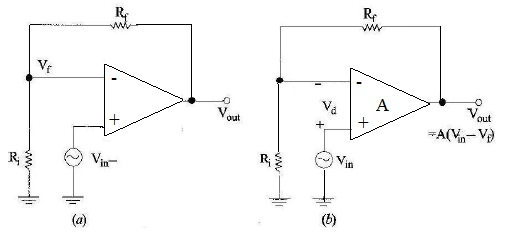Op-amp acts as both difference circuit and open-loop forward gain. Differential input to op-amp is (Vin - Vf). This differential voltage is amplified A times and output voltage is produced that is given by:

Vout = Av1 = A(Vin - Vf); where A is open-loop gain of op-amp. As, (Ri + Rf) acts as voltage divider across, Vout

If A is so large that 1 can be neglected as compared to βA, above equation becomes

A' = A/βA = 1/β = (Ri + Rf)/Ri

It is seen that closed-loop gain of the non-inverting op-amp is basically independent of open-loop gain.

Tutorsglobe: A way to secure high grade in your curriculum (Online Tutoring)

Expand your confidence, grow study skills and improve your grades.

Since 2009, Tutorsglobe has proactively helped millions of students to get better grades in school, college or university and score well in competitive tests with live, one-on-one online tutoring.

Using an advanced developed tutoring system providing little or no wait time, the students are connected on-demand with a tutor at www.tutorsglobe.com. Students work one-on-one, in real-time with a tutor, communicating and studying using a virtual whiteboard technology.  Scientific and mathematical notation, symbols, geometric figures, graphing and freehand drawing can be rendered quickly and easily in the advanced whiteboard.

Free to know our price and packages for online physics tutoring. Chat with us or submit request at info@tutorsglobe.com